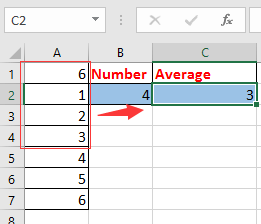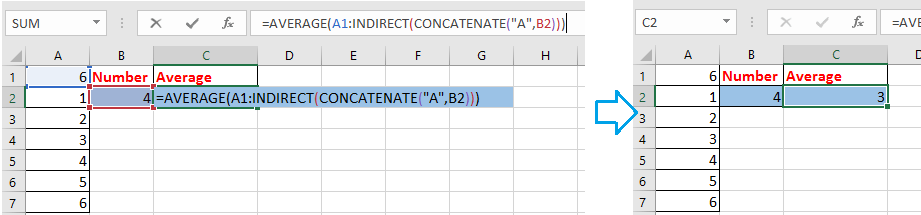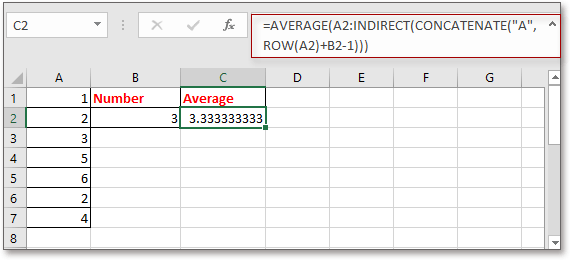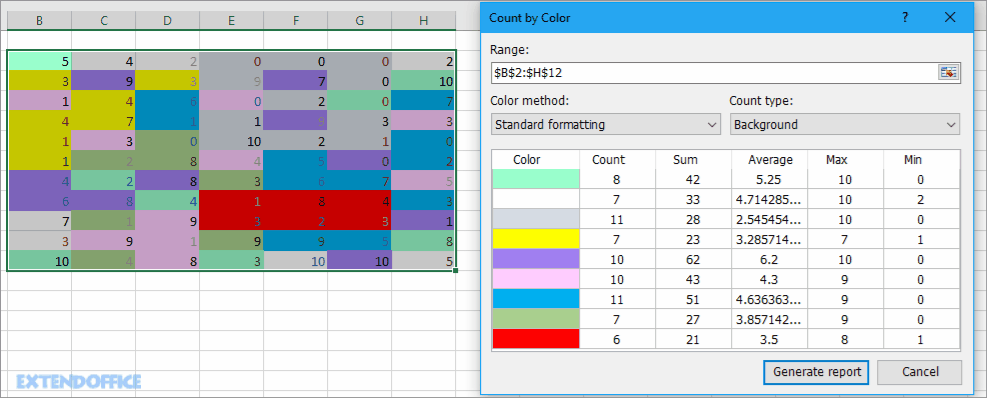## How to define range based on another cell value in Excel?

To calculate a range of values is easy for most of Excel users, but have you ever tried to calculate a range of values based on the number in a specific cell? For example, there is a column of values in column A, and I want to calculate the number of values in column A based on the value in B2, which means that if it is 4 in B2, I will average the first 4 values in column A as below screenshot shown. Now I introduce a simple formula to quickly define range based on another cell value in Excel.Define range based on cell value

####Define range based on cell value

To do calculation for a range based on another cell value, you can use a simple formula.

Select a blank cell which you will put out the result, enter this formula =AVERAGE(A1:INDIRECT(CONCATENATE("A",B2))), and press Enter key to get the result.Note:

1. In the formula, A1 is the first cell in the column you want to calculate, A is the column you calculate for, B2 is the cell you calculate based on. You can change these references as you need.

2. If you want to do summary, you can use this formula =SUM(A1:INDIRECT(CONCATENATE("A",B2))).

3. If the first data you want to define is not in first row in the Excel, for instance, in cell A2, you can use the formula as this: =AVERAGE(A2:INDIRECT(CONCATENATE("A",ROW(A2)+B2-1))).#### Quickly Count/Sum cells by background or format color in Excel

In some cases, you may have a range of cells with multiple colors, and what you want is to count/sum values based on same color, how can you quickly calculate?
With Kutools for Excel's Count by Color, you can quickly do many calculations by color, and also can generate a report of the calculated result.  Click for free full featured trial in 30 days!Kutools for Excel: with more than 300 handy Excel add-ins, free to try with no limitation in 30 days.

### Best Office Productivity Tools

Supports Office/Excel 2007-2021 and 365  |  Available in 44 Languages  |  Easy to Uninstall Completely

 Popular Features: Find/Highlight/Identify Duplicates   |  Delete Blank Rows   |  Combine Columns or Cells without Losing Data   |   Round without Formula ... Super Lookup: Multiple Criteria VLookup  |   Multiple Value VLookup  |   VLookup Across Multiple Sheets   |   Fuzzy Lookup .... Advanced Drop-down List: Quickly Create Drop Down List   |  Dependent Drop Down List   |  Multi-select Drop Down List .... Column Manager: Add a Specific Number of Columns   |   Move Columns   |   Unhide Columns   |   Compare Columns to Select Same & Different Cells ... Featured Features: Grid Focus   |  Design View   |   Big Formula Bar   |  Workbook & Sheet Manager   |  Resource Library (Auto Text)   |  Date Picker   |  Combine Worksheets   |  Encrypt/Decrypt Cells   |  Send Emails by List   |  Super Filter   |   Special Filter (filter bold/italic/strikethrough...) ... Top 15 Toolset:  12 Text Tools (Add Text, Remove Characters, ...)   |   50+ Chart Types (Gantt Chart, ...)   |   40+ Practical Formulas (Calculate age based on birthday, ...)   |   19 Insertion Tools (Insert QR Code, Insert Picture from Path, ...)   |   12 Conversion Tools (Numbers to Words, Currency Conversion, ...)   |   7 Merge & Split Tools (Advanced Combine Rows, Split Cells, ...)   |   Many More...

Kutools for Excel Boasts Over 300 Features, Ensuring That What You Need Is Just A Click Away...

Supercharge Your Spreadsheets: Experience Efficiency Like Never Before with Kutools for Excel  (Full-Featured 30-Day Free Trial)#### Office Tab Brings Tabbed interface to Office, and Make Your Work Much Easier

• Enable tabbed editing and reading in Word, Excel, PowerPoint, Publisher, Access, Visio and Project.
• Open and create multiple documents in new tabs of the same window, rather than in new windows.
• Increases your productivity by 50%, and reduces hundreds of mouse clicks for you every day!No ratings yet. Be the first to rate!
This comment was minimized by the moderator on the site
But If I want to sum a column range(say l7:l7800) out of the range d7:ct9000 based on the condition in column a(a7:a9000) and column b(b7:b9000). How will I do it. Please advise
This comment was minimized by the moderator on the site
Is there a way to create a dynamic ranged view, that will isolate all orders from a specific client within a list of clients? I have looked at multiple videos and none actually do what I need it to do. Is there a way to do this or would I need to pull the needed info into a controlled table and use the information from that table?
This comment was minimized by the moderator on the site
Hi, CareTaker. I do not get your question clearly. Could you describe your question or upload a file to show the quetion in details? But I recomend a utility called Data Association for you, maybe can help you, go to this web for details: https://www.extendoffice.com/product/kutools-for-excel/excel-click-cell-to-filter-based-on-another-workbook.html.
This comment was minimized by the moderator on the site
Thank you for the response, its quite the complicated thing to explain but I did manage to come right in the end using the indexing formula with the match formula as well.   =INDEX(\$B:\$B;MATCH(\$C\$2;\$B:\$B;0);):INDEX(\$N:\$N;MATCH(\$C\$2;\$B:\$B;0)+\$C\$3-1;)   at first it was confusing but I'm good now
This comment was minimized by the moderator on the site
how do I update sum range by using helper cell...For example: if initial sum range is = C1 to M1, how to do change sum range if new data is up to AB1 using a helper cell, instead of changing the formula manually?
This comment was minimized by the moderator on the site
What if the rage is not column, but a row?
This comment was minimized by the moderator on the site
That's a bit trickier as I just found out. You need to use a combination of the CHAR function to convert a number to a letter, CONCAT to piece together a letter and number comprising a cell address, and the INDIRECT function to recognize strings as cell addresses.

In my example, I wrote the following expression:

``````=SUM(INDIRECT(CONCAT("F",ROW())):INDIRECT(CONCAT(CHAR(64+5+\$B\$31),ROW())))
``````

where:
Row() is the current row
\$B\$31 is the cell where the # of columns to count is stored
5 is the offset for the first column to be counted

if you need to go beyond column Z, you’d need a less intuitive variant on that expression using the ADDRESS function:

``=SUM(indirect(concat("F",row())):indirect(ADDRESS(row(),\$B\$31+5,4)))``

where:
4 indicates a relative reference
This comment was minimized by the moderator on the site
Hi quick question. My formula =SUMIFS(DATA!\$D\$5:\$D\$13914,DATA!\$E\$5:INDIRECT(CONCATENATE("\$E\$",C3)),"<"&"1/1/2016") has a range that is from another tab called "DATA." I keep getting #VALUE! errors for this formula. Any suggestions? thanks!
This comment was minimized by the moderator on the site
Earlier I posted: Great formula but why does it only work when referring to the first cell. In other words, if you move the whole range down and the first cell in the range is A5 instead of A1, the formula ceases to work.
After playing with it, I figured out why the formula is not working when the starting row is not row 1. Excel interprets this as the range being A1 thru A4. If you are starting with a different row than row 1, here is how to modify the formula assuming the range now starts with A5:=MAX(A5:INDIRECT(CONCATENATE("A",ROW(A5)+B2)))
This works because the concatenation sees the row reference as fixed in the first example, but it becomes relative in the second example. Hope that helps!
Jared - You can just subtract 4 instead of adding. =MAX(A5:INDIRECT(CONCATENATE("A",ROW(A5)-B2))) [Assuming you have 4 in cell B2, or you could just leave the formula as is and put -4 in cell B2.]
This comment was minimized by the moderator on the site
Hi，cathy, thanks for your supplement, I have test your formula, I think the correct formula may be =MAX(A5:INDIRECT(CONCATENATE("A",ROW(A5)+B2-1))). I have update the tutorual. Thanks again.
This comment was minimized by the moderator on the site
Great formula but why does it only work when referring to the first cell. In other words, if you move the whole range down and the first cell in the range is A6 instead of A1, the formula ceases to work. That seems strange.
This comment was minimized by the moderator on the site
What if I want to count upward from a cell rather than downward? In the above example, the formula starts with A1 and goes downward. Let's say I want a range of 4 cells, beginning with Cell A10 and going to A6. Thank you!
This comment was minimized by the moderator on the site
Jared - See my most recent post for how to solve this.
This comment was minimized by the moderator on the site
I'm having this upward problem also but unable to see your other post for the solution. Can you give me link of the post please? Many thanks!
This comment was minimized by the moderator on the site
=AVERAGE(INDIRECT(CONCATENATE("A",COUNT(A:A)-B1+1)):INDIRECT(CONCATENATE("A",COUNT(A:A))))
A:A is the column that you want to calcualte the average values, B1 is the cell that define the range in column A to calculate.
This comment was minimized by the moderator on the site
Thanks, but may I know why need to use "-B1+1" please?
This comment was minimized by the moderator on the site
Hi, Let me take a detailed example to explain it for you. There are 10 rows in column A, I want to get the last 2 values' average, in other words, average the values in row 9 and row 10. Now B1 contains 2, =COUNT(A:A)-B1+1 will get the result 9, COUNT(A:A) will get the result 10 (the last row of the column A). Now combine other functions to averaget the values in row 9 and row 10. Hope this can help you.
This comment was minimized by the moderator on the site
Can I use this syntax while referring to a different sheet?
This comment was minimized by the moderator on the site
If you want to sum referring to a Different Shet In CONCATENATE formula write [ SHEET NAME+COLUMN+ROW]
Formula=SUM(INDIRECT(CONCATENATE("Sheet1!","D",1)):INDIRECT(CONCATENATE("Sheet1!","D",5)))
This comment was minimized by the moderator on the site
Hi, Thank you so much for your help, but I have a small problem that is I don't have just one column but several ones (about 100 columns) is there a way to change the formula to accommodate the change in columns so O can get the sum for Columns A B C and so on. thanks for your help
This comment was minimized by the moderator on the site
Thank u for your message. If you want to sum first n values across multiple continuous columns, just change A to the last column you ues. EG, sum first 5 values across column A to column D, apply this formula =SUM(A1:INDIRECT(CONCATENATE("D",F2))).
There are no comments posted here yet